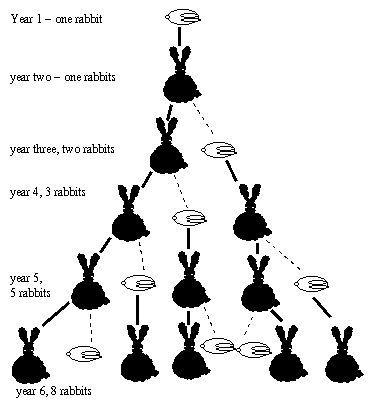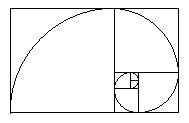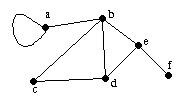Applications of linear algebra

I'd like to look at applications today, but first we will have to go over the projections that we still didn't finish properly in the last lecture. We will look briefly at the applications sections of chapter 3, but we will not look at the section on partitioned matrices, since this section is not very difficult once you've got the hang of matrix multiplication, so if you do find you need it at a later stage, it should be easy to pick up. Anyone who is especially keen on that section can always work through it on their own and do it for their project.

Applications of matrices

We will look at the following:
• Markov chains
• Fibonnaci numbers
• Graphs and games

If there was more time, we'd look at all of the above in more detail, but we only have time to have a quick look.

Markov chains

We will look at the example of population movement, page 87 of text book. See also page 259.
This example was done in lecture 6, so I will not say much about it in this class, just mention a few other examples the book deals with, and which some of you may like to write about for your project.

Fibonnaci sequence

The Fibonnaci sequence looks like:
1,1,2,3,5,8,13,21,34,...

To get the next number, you add the previous two. This is an important sequence in mathematics, in nature, perhaps even in art.
It was first discovered in connection with rabbits...
We begin with one baby rabbit. When a rabbit is a year old, it can start reproducing, and then it has a baby every year. In the picture below, the baby rabbits are the ones lying down. The thick lines mean the rabbits joined are the same rabbit, just a year older. The dashed lines means that one rabbit is the other's baby.
How many rabbits will there be after 30 years? (These rabbits live forever.)It comes up in lots of other places, eg, in the following spiral, which is the shape of many sea shells, the squares drawn have side length successive Fibonnaci numbers:What has this got to do with matrix multiplication?
Quite a lot. Try multiplying the following matrix by itself several times, and see what happens:
 / 1 1 \ \ 1 0 /
If you could find this matrix to the power n, you could find how many rabbits there are after n years, or how big the spiral is after n steps. When we've looked at diagonalization, this will be a much easier problem. Even without diagonalization, using matrices makes things easier. Is it any faster to find the 32nd Fibonnaci number now?

Although matrices are generally for studying linear systems, some non linear things have linear aspects, and can be studied with matrices.
A quadratic form is an equation where everything has degree 2:
Eg: x^2+3y^2+7xy

As graphs, we get things like circles and ellipses, and hyperbolas.
These things can be described by matrices. To see how, find the following product:
 ( x y ) / a b \ / x \ \ c d / \ y /

So, we can use methods of linear algebra to look at quadratic forms.
Section 8.2 of the text book does this in more detail.

Graphs

In this context, a graph is a collection of points ("vertices"), joined by lines ("edges"). eg:Graphs have many applications, eg, what's a good way to connect together lots of computers in a good way.
Matrices are a very useful way of studying graphs, since they turn the picture into numbers, and then we can calculate, and use linear algebra techniques.
To represent the above graph by a matrix, make a table, and put a 1 to mean those points are conenected by a line, and a zero if they are not:
a b c d e f
a 1 1 0 0 0 0
b 1 0 1 1 1 0
c 0 1 0 1 0 0
d 0 1 1 0 1 0
e 0 1 0 1 0 1
f 0 0 0 0 1 0

Calculate the square of the matrix:
 / 1 1 0 0 0 0 \ / 1 1 0 0 0 0 \ / 2 1 1 1 1 0 \ | 1 0 1 1 1 0 | | 1 0 1 1 1 0 | | 1 4 1 2 1 1 | | 0 1 0 1 0 0 | | 0 1 0 1 0 0 | = | 1 1 2 1 2 0 | | 0 1 1 0 1 0 | | 0 1 1 0 1 0 | | 1 2 1 3 1 1 | | 0 1 0 1 0 1 | | 0 1 0 1 0 1 | | 1 1 2 1 3 0 | \ 0 0 0 0 1 0 / \ 0 0 0 0 1 0 / \ 0 1 0 1 0 1 /

This tells us about how well points are connected over a distance of 2 lines. Eg, there are 2 ways to get from b to d in two steps; but there are no ways to get from a to f in two steps.

A graph theory game

The game is that there are 4 connected dots, which are lights. If you click a dot, it changes whether it is off or on, and so do all the dots which are joined to it by lines. The aim is to turn all the lights on.
See if you can do it for the following:
 - | | -

(This is written in Java script; it works on the computer I'm using now; I don't know if it works on all computers. Maybe if you are better at Java script than me you can help me write the code in a better way.)
Can you get them all turned on at once, and then all off again. Does it make any difference which order you make your clicks? Are there different ways to get to all on or all off?
What if we start from a different position?
What if we add more connections?
What if we start from a different graph?
Or this:
 - -
Or this:
 -- \ /

Can you solve the puzzle? How is linear algebra involved?
The answer is that you need to find the inverse of the matrix of the connections. How this worked was explained in class. We also had to use "mod 2" arithmetic, that is 1+1=0 when we're thinking about 1 meaning switch once; switching twice is the same as switching zero times.
For example, for the first of the above graphs, we can lable the lights a,b,c,d:
 a - b | | c - d

We start with them all off, so if sa is the state of a, sb the state of b, etc, and the state can be either 0 for off or 1 for on, then we start with:
(sa,sb,sc,sd)=(0,0,0,0)

We want to end up with
(sa,sb,sc,sd)=(1,1,1,1)

So the change we want to make is (1,1,1,1) (meaning change everything). (If we'd started with (1,1,0,0), we'd only want to make the change (0,0,1,1), meaning only change the last two).
If A is the matrix of the graph, which describes how clicking one button affects all the buttons, then if v is a vector describing which buttons you click, eg (1,0,0,1) would mean click a and d, but not b and c, then the change you get will be the vector Av. We want
Av=(1,1,1,1)

So v will be given by
v=A-1(1,1,1,1)
(note, the vectors should have been drawn vertically).
In our case, A is given by
 / 1 1 1 0 \ | 1 1 0 1 | | 1 0 1 1 | \ 0 1 1 1 /

So to find how to make whatever change in the lights you want, you just need to be able to find the inverse of A, using mod 2 arithmetic. (Which is like ordinary arithmetic, except whenever you get an even number, you write 0 instead, and odd numbers can always be replaced by 1.)
This is a very brief outline of the solution. For more details, see the following references:
• Problem solving using geometry games, by Ole Bjorkqvist. (This is probably the best reference for an introductory approach; unfortunately I'm not sure where I got it from.)
• The sigma game and Cellular Automata, by Klaus Sutner, American Math Montly, Jan 1990, Vol 97, #1
• Linear cellular automata and the Garden of Eden, by Klaus Sutner, The Mathemaitcal Intelligencer Vol. 11, No. 2, 1989

Assignment

To be handed in on Tuesday 3rd June.
• section 6.1: 6, 16, 20, 22, 24, 26, 32
• section 6.2: 8, 10, 16
• section 6.3: 2, 4, 6, 12, 22

What will be on the quiz

On Thursday 29th of May there will be a quiz, with a question like the following:
Do the vectors (1,0,5), (1,2,5) and (1,3,1) span R3?
Find the inverse of an appropriate matrix to find the solutions to the following problems:
a1(1,0,5)+a2(1,2,5)+a3(1,3,1)=(1,0,0)

b1(1,0,5)+b2(1,2,5)+b3(1,3,1)=(0,1,-1)

c1(1,0,5)+c2(1,2,5)+c3(1,3,1)=(0,0,2)

Solution:

To ask if we can span R3 with these vectors means can we get to any point in R3 using them, eg, how can we get to (x,y,z) for any choice of x,y,z. This means asking if the following vector equation has a solution:
 / 1 \ / 1 \ / 1 \ / x \ a | 0 | + b | 2 | + c | 3 | = | y | \ 5 / \ 5 / \ 1 / \ z /
Solving this is the same as solving the matrix vector equation:
 / 1 1 1 \ / a \ / x \ | 0 2 3 | | b | = | y | \ 5 5 1 / \ c / \ z /

if a=(a,b,c), and x=(x,y,z) (strictly speaking, I should say a is the transpose of (a,b,c), to make it vertical) (note, a is not the same as a - one is a vector, the other is a number). Suppose the matrix is called A. The the above equation is
Aa=x

This equation tells us how to get from knowing (a,b,c) to knowing (x,y,z). But we want to know the reverse. We are told (x,y,z), and we want to find (a,b,c). The transformation that will do that for us, if it exists, will be the inverse of A, and we'll have
a=A-1x

So, if we can find an inverse for A, then the vectors do span R3, and then we can use A-1 to solve the problems of how to get to the three given vectors, (1,0,0), (0,-1,-1), and (0,0,2).
The inverse is found as follows, using row reduction, as described in class:
 / 1 1 1 | 1 0 0 \ / 1 1 1 | 1 0 0 \ / 1 1 1 | 1 0 0 \ | 0 2 3 | 0 1 0 | | 0 2 3 | 0 1 0 | | 0 2 3 | 0 1 0 | \ 5 5 1 | 0 0 1 / R3-5R1 \ 0 0 -4 | -5 0 1 / -R3/ 4 \ 0 0 1 | 5/4 0 -1/4 /

 / 1 1 1 | 1 0 0 \ / 1 1 1 | 1 0 0 \ R1-R3 / 1 1 0 | -1/4 0 1/4 \ R2-3R3 | 0 2 0 | -15/4 1 3/4 | R2/2 | 0 1 0 | -15/8 1/2 3/8 | | 0 1 0 | -15/8 1/2 3/8 | \ 0 0 1 | 5/4 0 -1/4 / \ 0 0 1 | 5/4 0 -1/4 / \ 0 0 1 | 5/4 0 -1/4 /

 R1-R2 / 1 0 0 | 13/8 -1/2 -1/8 \ | 0 1 0 | -15/8 1/2 3/8 | \ 0 0 1 | 5/4 0 -1/4 /

so the inverse matrix is:
 / 13/8 -1/2 -1/8 \ | -15/8 1/2 3/8 | \ 5/4 0 -1/4 /

(Check with MatLab!)
Now we can solve the problems:
 / 13/8 -1/2 -1/8 \ / 1 \ / 13/8 \ | -15/8 1/2 3/8 | | 0 | = | -15/8 | \ 5/4 0 -1/4 / \ 0 / \ 5/4 /

 / 13/8 -1/2 -1/8 \ / 0 \ / -3/8 \ | -15/8 1/2 3/8 | | 1 | = | 1/8 | \ 5/4 0 -1/4 / \ -1 / \ 1/4 /

 / 13/8 -1/2 -1/8 \ / 0 \ / -1/4 \ | -15/8 1/2 3/8 | | 0 | = | 3/4 | \ 5/4 0 -1/4 / \ 2 / \ -1/2 /

The solutions are
a1=13/8, a2=-15/8, a3=5/4, b1=-3/8, b2=1/8, b3=1/4, c1=-1/4, c2=3/4, c3=-1/2,

Lecture 6 Back to the Linear Algebra Notes Index. Lecture 8